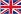(+86)-029-85200606       sales@guphe.comEnglish

# Three methods for calculating the heat transfer area of a plate heat exchanger

Views:1     Author:Site Editor     Publish Time: 2020-05-29      Origin:Site

## (I) Mean temperature difference method

According to the basic equation of heat transfer, the required heat transfer area can be obtained as

F = Q / K. Δtm

Tip: Q—heat flow (W), △ tm—logarithmic average temperature difference (℃), F—heat transfer area (m2)

### (2) Number of heat transfer units

The number of heat transfer units is a parameter that reflects the ease of heat exchange between the cold and hot fluids, and is also a parameter that measures the heat transfer capacity of the heat exchanger. The definition of the number of heat transfer units NTU can be more widely expressed as

(NTU 1) = KA / C 1 or (NTU 2) = KA / C 2 = γ 1 (NTU 1)

Where C 1 and C 2 are the heat capacities of hot and cold fluids, respectively.

Obviously, as long as the NTU, C and the total heat transfer coefficient K are known, the heat transfer area can be obtained from.

The number of heat transfer units is related to the temperature efficiency ε and the ratio γ of the heat capacity of the Liang Huan fluid. The temperature efficiency ε is the ratio of the temperature change of any fluid participating in the heat exchange to the temperature difference between the inlets of the cold and hot fluids.

ε 1 = (t 1′-t 1 ″) / (t 1′-t 2 ′)

Or ε₂ = (t ₂ ″ -t ₂ ′) / (t ′ ′ -t ₂ ′) = γ ε ε

Where the heat capacity ratio γ is

γ 1 = C 1 / C 2 or γ 2 = C 2 / C 1 = 1 / γ 1

By establishing an energy balance method, the relationship between the temperature efficiency, the number of heat transfer units, and the ratio of heat capacity can be obtained.

(3) Calculation of heat transfer area after the process combination is determined

Regardless of whether the average temperature difference method or the NTU method is used, a process combination must be set to calculate the plate exchange heat exchange area. The calculated heat exchange area and the heat exchange area of the process combination are equal or slightly less than the working conditions , Otherwise, a process combination should be set and calculated again until the working conditions are met.

Form Name